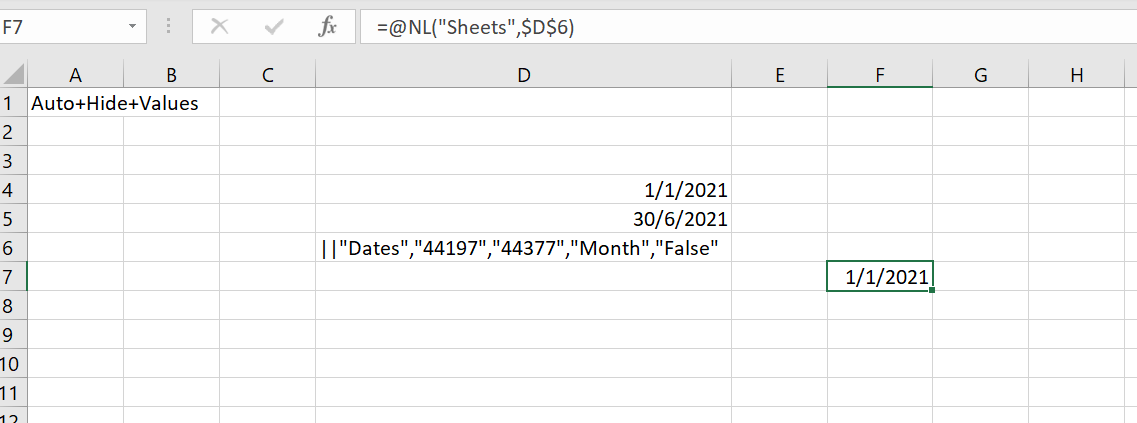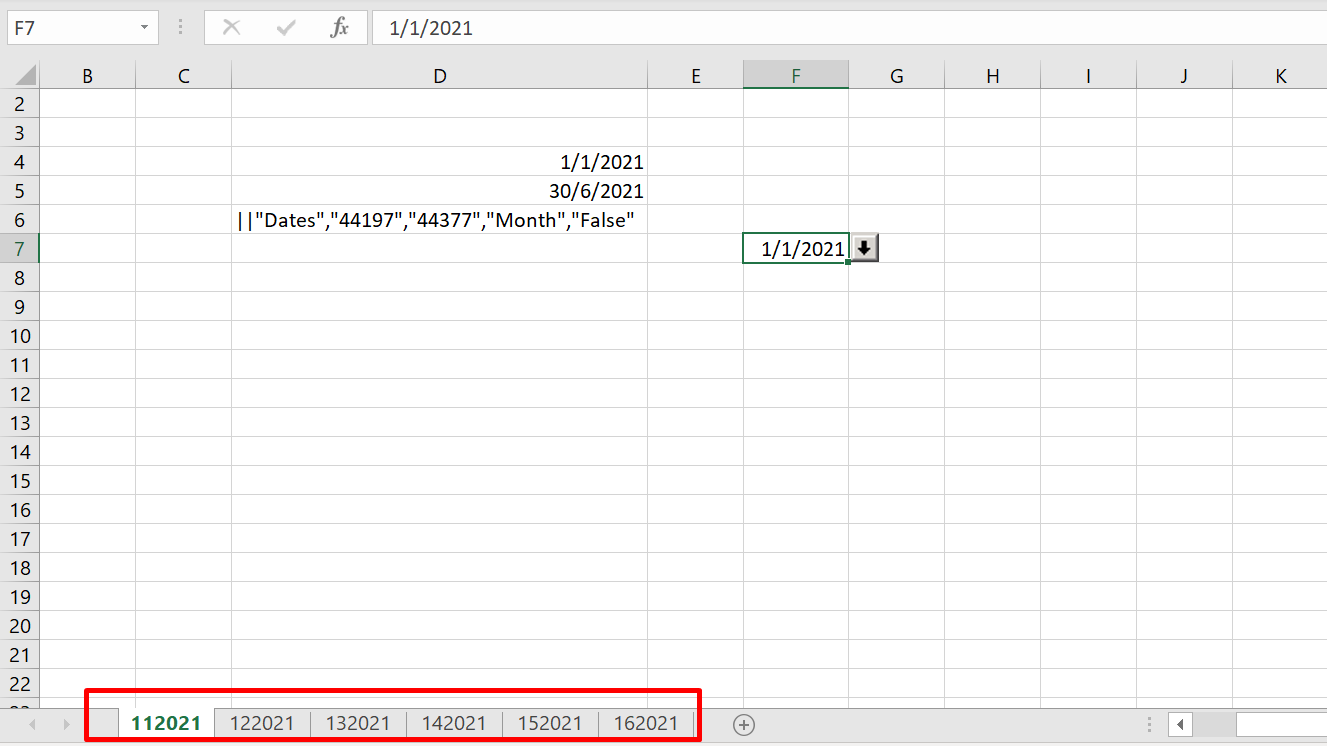Hi,

I would like to change the sheet name after run the report, using NP Dates function + NL Sheet. Anyone know how can I get the sheet in MM YYYY format?•BryanAtTigunia

This is fairly complex, if I remember correctly because you have to use Excel to extract the month and year from the date field from the Date table for the sheet name but then you have to use Excel to re-convert back to a date on each individual sheet.

Might look something like using a NAV/BC data source...

=NL("sheets","date","=text(month(NF(,""Start Date""),""00"")&text(year(NF(,""Start Date""),""0000"")))","Start Date",\$d\$4,"End Date",\$D\$5)

...to generate the sheet name.  I don't have a way to test so this may not be 100% accurate but at least it shows that it isn't just a quick solution.

To extract the start date in a date format for the report, you would probably have to do something like =DATE(RIGHT(F7,4),LEFT(F7,2),1) where F7 is the value of MM YYYY

I'm not entirely sure how I would do that using NP("Dates")

Edited by BryanAtTigunia
•Neal Carter

Found a simple way to do this using OFFSET.

=NL("Sheets",TEXT(OFFSET(Periods,0,1,NoPeriods,1),"mmm yy")) where Periods is a range of dates like 01/04/2023, 01/05/2023, etc. and NoPeriods is the number of periods required.

That will create the sheets.

Extract the date from the formula for use on each sheet using =DATEVALUE(D4) where D4 is the sheets formula.

Now you have sheets named Apr 23, May 23, Jun 23, etc. but still reference the start date with the 2nd formula.